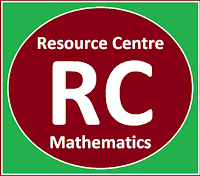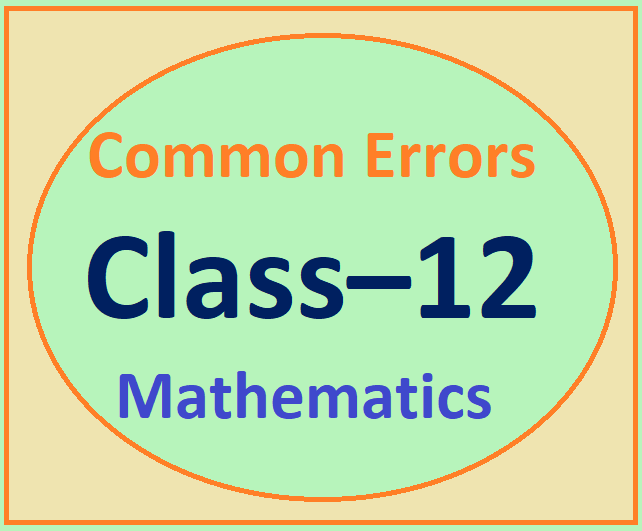## Posts

Showing posts from July, 2022

### Resource Centre Mathematics

Resource Centre Mathematics Mathematics worksheet, mathematics basic points and formulas, mathematics lesson plan, mathematics multiple choice questions Workplace Dashboard CBSE Syllabus For Session 2023-24 For  :   Classes IX & X    |   Classes XI & XII Watch Videos on Maths Solutions CLASS IX MATHEMATICS FORMULAS &  BASIC CONCEPTS

### Math Class 11 Ch-1 NCERT Sol. | Miscellaneous ExerciseClass 11  Chapter -1 Set Theory Miscellaneous Exercise NCERT solution of chapter 1, class 11, miscellaneous exercise, Union of sets, intersection of sets, complement of sets and Venn diagram of sets, word problems on sets. Q1) A = {x : x ∈ R and x satisfy x 2 -8x+12 = 0} B = {2, 4, 6},  C = {2, 4, 6, 8, ....}, D = {6} Solution: x 2  - 8x + 12 = 0 ⇒ x 2  - 6x - 2x + 12 = 0 ⇒ x(x - 6) -2(x - 6) = 0 ⇒ (x - 2)(x - 6) = 0 ⇒ x = 2, x = 6 ⇒ A = {2, 6} A ⊂ B,  A ⊂ C, B ⊂ C, D ⊂ A,  D ⊂ B,  D ⊂ C Q2) (i) If x ∈ A and A∈B, then x ∈ B Ans:  False Statement Reason: If A = {1,2}, B={{1,2}, 3} Here 1∈A and A ∈ B but 1 ∉ B So given statement is false (ii) If A ⊂ B and B ∈ C, then A ∈ C Let A = {1}, B={1, 2}, C ={{1, 2}, 3} Here  A ⊂ B  and B ∈ C,  But 1∉ C So this is a false statement (iii) If A ⊂ B and B ⊂ C, then A ⊂ C Ans: True Statement Explanation:  Let A={1}, B={1,2}, C = {1,2,3} Here  1 ∈ A and 1 ∈ B  ⇒ A  ⊂ B .... (1) 1, 2 ∈ B and 1,2 ∈ C  ⇒ B  ⊂ C ....... (2) From (1) and (2) we get  A ⊂ C

### Resource Centre MathematicsResource Centre Mathematics Mathematics worksheet, mathematics basic points and formulas, mathematics lesson plan, mathematics multiple choice questions Workplace Dashboard CBSE Syllabus For Session 2023-24 For  :   Classes IX & X    |   Classes XI & XII Watch Videos on Maths Solutions CLASS IX MATHEMATICS FORMULAS &  BASIC CONCEPTS

### Common Errors in Class 12 MathematicsCOMMON ERRORS DONE BY STUDENTS   Class 12, Mathematics:  Common errors or common mistakes done by students while solving the questions in the examination, Common mistakes done by the students of class XII while solving the mathematical problems. Chapter 1 Relations and Functions